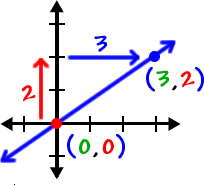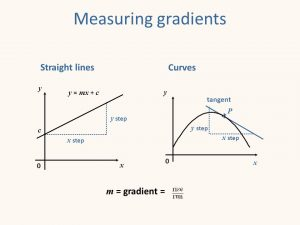# Kinematics Part 1

## Choosing the Right Path…

Kinematics is the study of motion of a body in question. And very often, we are concerned about its displacement, velocity, and acceleration. The aforementioned equations involve the use of more than one of these quantities, but before we get on to the specifics of each equation, it is important that you know what each quantity means.

##### Defining displacement (s)

Displacement is the change of displacement from the origin. If you move from the origin to 5, your new displacement from the origin is 5. If you move from 5 to 8, your new displacement FROM the origin is 8.

##### Defining velocity (v)

Velocity is the rate of change of position. Mathematically, we can say v = Δs/Δt.

What does the Δ mean? It is the Greek letter delta, and it means “change in”.  Simply put, velocity is (change in displacement) / (change in time). Taking the previous numerical example, if you move from 5 to 8, your new displacement FROM the origin is 8, but the change in your displacement is 3.

The same logic about Δ holds true for time. We imagine that there is a clock that keeps track of the time in each problem. If we have motion that starts at t = 5 and ends at t=9, it is important to recognize that the change in t is 4, and that’s what we care about if we are calculating the velocity during the period from t = 5 to t = 9.

Velocity is the slope (or gradient) of the displacement vs time graph

Note that in a s-t graph, then Δs/Δt is the slope (also called the gradient) of that graph, and we can find velocity by calculating that slope.  Δs is the rise, and Δt is the run.In the picture above, the RISE is 2 units and RUN is 3 units. A simple calculation will give you a slope of 3/2.

If the s-t graph is a straight line, velocity is constant, because a straight line has a constant slope.  If the s-t graph is not a straight line, we can still find the velocity at any particular instant in time by drawing a tangent line to the curve at that point and measuring its slope.It is important for you to understand that velocity is the slope of the displacement-time graph.  Think about it and make some sketches of graphs for yourself until you really get it.

Click here for an article for a more detailed understanding of velocity as the slope of displacement vs time

##### Defining acceleration

Acceleration is rate of change of velocity. Note that an object that is undergoing acceleration doesn’t necessarily mean that it is speeding up. If you are in your car going straight at 20 km/hr, and you speed up to 30 km/hr, your velocity changed by 10 km/hr. If you made that change in 5 seconds, then your acceleration was (10 km/hr) / ( 5 seconds) = (2 km/hr)/s. We can write a = Δ v / Δ t. If the change in velocity is negative, then you have negative acceleration. Negative acceleration could mean you are slowing down, and we still call that acceleration. But it could also mean that you are speeding up in the negative direction!

Acceleration is the gradient (or slope) of the velocity vs time graph

Just like velocity was the slope of displacement, because v = Δs/Δt, so too is acceleration the slope of velocity, because a = Δv/Δt.  Δv is the rise and Δt is the run.  If velocity is increasing at a steady rate, then acceleration will be a positive constant.  If velocity is decreasing at at steady rate, then acceleration will be a negative constant  If velocity is changing, then acceleration is constant at 0.  Obviously you can go the other way, too:  if acceleration is a positive constant, then the velocity graph is a straight line tilting up; if acceleration is a negative constant, then the acceleration graph is a straight line tilting down, and if acceleration is 0, the velocity graph must be flat. Be sure not to confuse zero acceleration with zero velocity.  Zero acceleration means velocity is not changing.  It could be 0 and not changing, but it could also have any non-zero value without changing.

Do you still need help with acceleration concepts? Try this video

The kinematic equations

Teachers often open this topic by introducing students to the four essential equations in solving questions related to the topic. And if you need a recap of what they are, we have them all listed out for you:

1. s = (u + v)*t/2
2.  v = u + at.
3.  s = ut + 1/2*a*t^2
4. v^2 = u^2 + 2as.

Committing these equations to memory is one thing, knowing how to apply them is another. But that’s still not enough. Physics is not about just figuring out how to apply formulas like a robot. There’s nothing wrong with using the formulas, but you need to understand what each one is saying and why it works. When you do, you will almost always quickly see more than one method to solving any kinematics problem.

It is very important to remember that these equations only apply for uniform (constant) acceleration. Constant acceleration can include zero acceleration, of course.

Now…

Where do the equations come from?

We’ll admit that knowing the derivation of these equations may not be very helpful in solving problems, but knowing one in particular will be helpful in dealing with subsequent topics. The equation Δs = ut + 1/2*a*t^2 is derived from finding the  area under the curve of the v-t graph. Know for sure that you are going to see this same area-under-the-curve approach used again and again, for example, in the topics work energy power, gravitation, etc…

If you don’t see why displacement is the area under the velocity vs time curve, this video will help, and you should watch it, because this is a really important and useful concept that we apply over and over in

Using area under the velocity vs time curve to find total displacement and average velocity (KA video)

Using area under the acceleration vs timo)